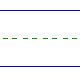Name: ______________________________________________ Date: _____________

Division

Instructions: Divide.

 16 ÷ 2 =0 ÷ 3 =3 ÷ 1 =8 ÷ 2 =0 ÷ 6 =0 ÷ 5 =6 ÷ 2 =8 ÷ 1 =1 ÷ 1 =7 ÷ 1 =2 ÷ 1 =0 ÷ 2 =0 ÷ 8 =9 ÷ 1 =4 ÷ 1 =12 ÷ 2 =0 ÷ 4 =0 ÷ 7 =